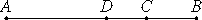# Proposition 43

A first bimedial straight line is divided at one and the same point only.
X.37

Let AB be a first bimedial straight line divided at C, so that AC and CB are medial straight lines commensurable in square only and containing a rational rectangle.

I say that AB is not so divided at another point.

If possible, let it also be divided at D, so that AD and DB are also medial straight lines commensurable in square only and containing a rational rectangle.X.26

Since, then, that by which twice the rectangle AD by DB differs from twice the rectangle AC by CB is that by which the sum of the squares on AC and CB differs from the sum of the squares on AD and DB, while twice the rectangle AD by DB differs from twice the rectangle AC by CB by a rational area, for both are rational, therefore the sum of the squares on AC and CB also differs from the sum of the squares on AD and DB by a rational area, though they are medial, which is absurd.

Therefore a first bimedial straight line is not divided into its terms at different points. Therefore it is so divided at one point only.

Therefore, a first bimedial straight line is divided at one and the same point only.

Q.E.D.

## Guide

This proposition is not used in the rest of the Elements.